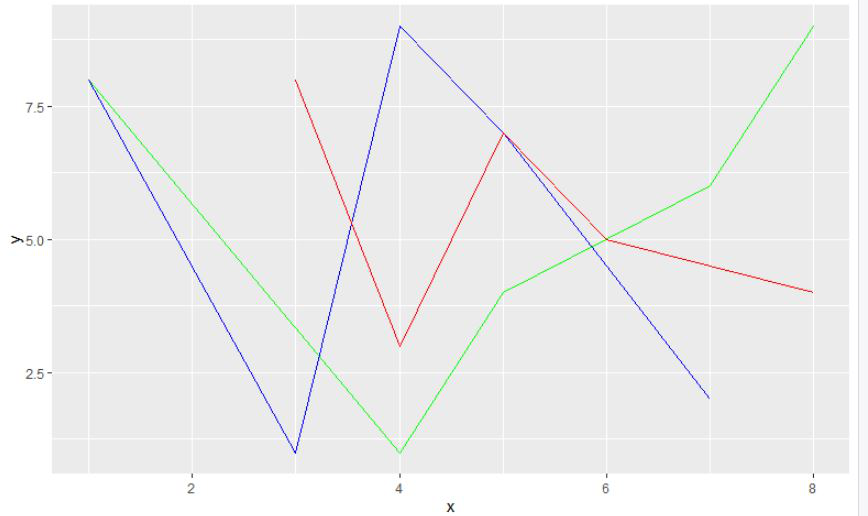Open in App
Not now

# How to Combine Multiple ggplot2 Plots in R?

• Last Updated : 28 Nov, 2021

In this article, we will discuss how to combine multiple ggplot2 plots in the R programming language.

## Combining multiple ggplot2 plots using ‘+’ sign to the final plot

In this method to combine multiple plots, here the user can add different types of plots or plots with different data to a single plot, the user only needs to use the ‘+’ sing to combine the plot with each plot to the final plot, and further, the final plot will be a combination of multiple plots in the single ggplot2 plot in the R programming language.

Syntax:

ggplot(NULL, aes(x, y)) +geom_line(data 1)+geom_line(data 2)+……+geom_line(data n)

Example 1:

In this example, we will be combining 3 ggplot2 line plots with different data using the ‘+’ sign form combining them into a single plot in the R programming language.

## R

 `# load the package``library``(``"ggplot2"``)`` ` `# create dataframe``gfg1 < -``data.frame``(x=``c``(4, 5, 1, 7, 8), ``                   ``y=``c``(1, 4, 8, 6, 9))`` ` `# create dataframe``gfg2 < -``data.frame``(x=``c``(5, 1, 4, 3, 7),``                   ``y=``c``(7, 8, 9, 1, 2))`` ` `# create dataframe``gfg3 < -``data.frame``(x=``c``(4, 8, 6, 3, 5),``                   ``y=``c``(3, 4, 5, 8, 7))`` ` `# plot the data with dataframes with green``# red and blue colors``gfg_plot < -``ggplot``(``NULL``, ``aes``(x, y)) + ``geom_line``(data=gfg1, col=``"green"``) +``geom_line``(data=gfg2, col=``"blue"``)+``geom_line``(data=gfg3, col=``"red"``)`` ` `# display the plot``gfg_plot`

Output:Example 2:

In this example, we will be combining 3 ggplot2 line plots and 2 scatter ggplot2 plots a total of 5 plots with different data using the ‘+’ sign form combining them into a single plot in the R programming language.

## R

 `# load the package``library``(``"ggplot2"``)`` ` `# create 5 dataframes``gfg1 < -``data.frame``(x=``c``(4, 5, 1, 7, 8), ``                   ``y=``c``(1, 4, 8, 6, 9))``gfg2 < -``data.frame``(x=``c``(5, 1, 4, 3, 7),``                   ``y=``c``(7, 8, 9, 1, 2))``gfg3 < -``data.frame``(x=``c``(4, 8, 6, 3, 5), ``                   ``y=``c``(3, 4, 5, 8, 7))``gfg4 < -``data.frame``(x=``c``(8, 6, 7, 2, 1), ``                   ``y=``c``(8, 6, 4, 1, 9))``gfg5 < -``data.frame``(x=``c``(6, 1, 6, 5, 4),``                   ``y=``c``(6, 8, 7, 6, 4))`` ` `# plot the data with 5 dataframes``# with 5 different colors``gfg_plot < -``ggplot``(``NULL``, ``aes``(x, y)) + ``geom_line``(data=gfg1, col=``"green"``) +``geom_line``(data=gfg2, col=``"blue"``)+``geom_line``(data=gfg3, col=``"red"``)+``geom_point``(data=gfg4, col=``"purple"``) + ``geom_point``(data=gfg5, col=``"black"``)`` ` `# display the plot``gfg_plot`

Output:My Personal Notes arrow_drop_up